Next: Relation to K-means clustering. Up: Self-organizing maps Previous: Outliers.

### Mathematical characterizations

Rigorous mathematical treatment of the SOM algorithm has turned out to be extremely difficult in general (reviews have been provided by Kangas, 1994; and Kohonen, 1995c). In the case of a discrete data set and a fixed neighborhood kernel, however, there exists a potential function for the SOM, namely [Kohonen, 1991, Ritter and Schulten, 1988]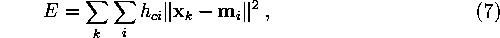where the index c depends on the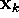and the reference vectors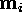(cf. Eq. 5).

The learning rule of the SOM, Equation 6, corresponds to a gradient descent step in minimizing the sample function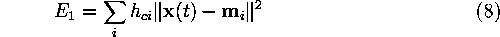obtained by selecting randomly a sample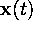at iteration t. The learning rule then corresponds to a step in the stochastic approximation of the minimum of Equation 7, as discussed by Kohonen (1995c).

Note: In Equation 7 the index c is a function of all the reference vectors, which implies that it may change when the gradient descent step is taken. Locally, if the index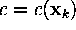does not change for any, the gradient step is valid, however.

Sami Kaski
Mon Mar 31 23:43:35 EET DST 1997